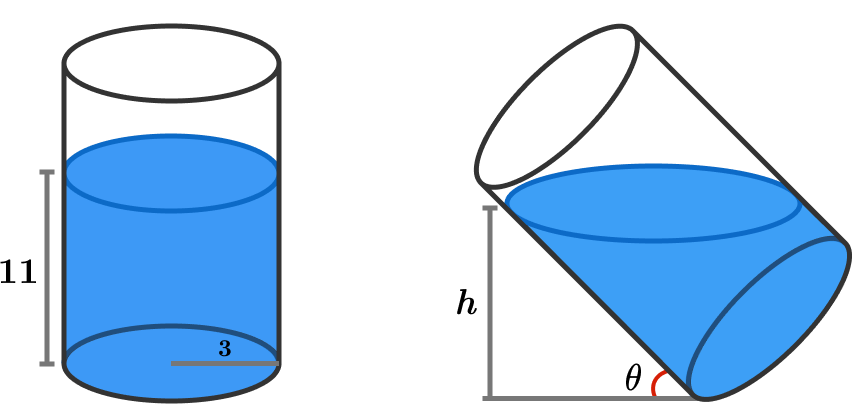# Don't Spill it!

Geometry Level 4A cylindrical glass of radius 3 is filled with water until its height reaches 11, as shown above left.

Now, if the cylinder is tilted such that it forms an angle of $\theta$ with the ground with $\cos \theta = \frac{4}{5}$, what is the new height $h$?


Clarification: The cylinder is so long that no water spills over.

×